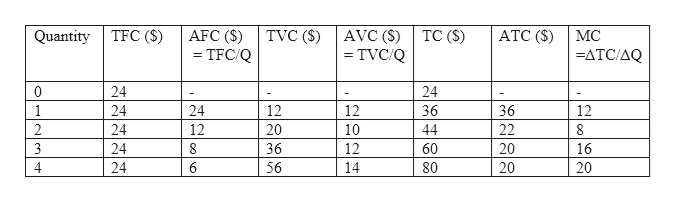# Draw a graph and complete a short-run cost table by using the information provided.      (TP)   (TFC)     (AFC)    (TVC)    (AVC)      (ATC)      (TC)         (MC)       0        \$          \$          \$         \$             \$            \$             \$       1                                           \$12       2                    \$12                 \$10        3                                           \$12       4                                           \$14

Question
141 views

Draw a graph and complete a short-run cost table by using the information provided.

(TP)   (TFC)     (AFC)    (TVC)    (AVC)      (ATC)      (TC)         (MC)

0        \$          \$          \$         \$             \$            \$             \$

1                                           \$12

2                    \$12                 \$10

3                                           \$12

4                                           \$14

check_circle

Step 1

In the short run, a firm will have two types of cost: fixed cost and variable cost.

Total fixed cost (TFC): The cost of all the fixed inputs in a production process is the total fixed cost. This cost does not change with the quantity of output produced.

Total variable cost (TVC): The cost of all the variable inputs in a production process is the total variable cost. For example, the cost of hiring labor and maintenance cost are variable costs. This cost changes with the quantity of output produced.

Step 2

Total cost of production for a firm is the sum of total fixed cost and total variable cost.

TC = TFC + TVC

The 6th column of table that is value of total cost is calculated by adding the values of total fixed cost and total variable cost.

Marginal cost: It is the additional cost of production when one more unit of quantity is produced.

MC = ΔTC/ΔQ

In the table, MC is calculated as:

If TC at q = 1 is 36

TC at q = 2 is 44

Change in quantity ΔQ = 2-1 = 1

Change in total cost ΔTC = 44 – 36 = 8

Therefore, MC = ΔTC/ΔQ

= 8/1 = 8

With same method, the remaining values of marginal costs can be calculated.help_outlineImage TranscriptioncloseTFC (\$) AFC (S) = TFC/ Q TVC (S) TC (S) ATC (S) Quantity AVC (S) -TVC/Q MC =ATC/AQ 0 24 24 1 24 24 12 12 36 36 12 2 24 12 20 10 44 22 8 3 24 8 36 12 60 20 16 4 24 6 56 14 80 20 20 fullscreen
Step 3

Average variable cost (AVC): AVC is the variable cost per unit of output. It can be express as:

AVC = TVC/Q

For example, from the table when quantity is 4 and total variable cost TVC is 56 then AVC is:

AVC = TVC/Q = 56/4 = 14.

Average fixed cost (AFC): AFC is the fixed cost per unit of output. It can be express as:

AFC = TFC/Q...

### Want to see the full answer?

See Solution

#### Want to see this answer and more?

Solutions are written by subject experts who are available 24/7. Questions are typically answered within 1 hour.*

See Solution
*Response times may vary by subject and question.
Tagged in